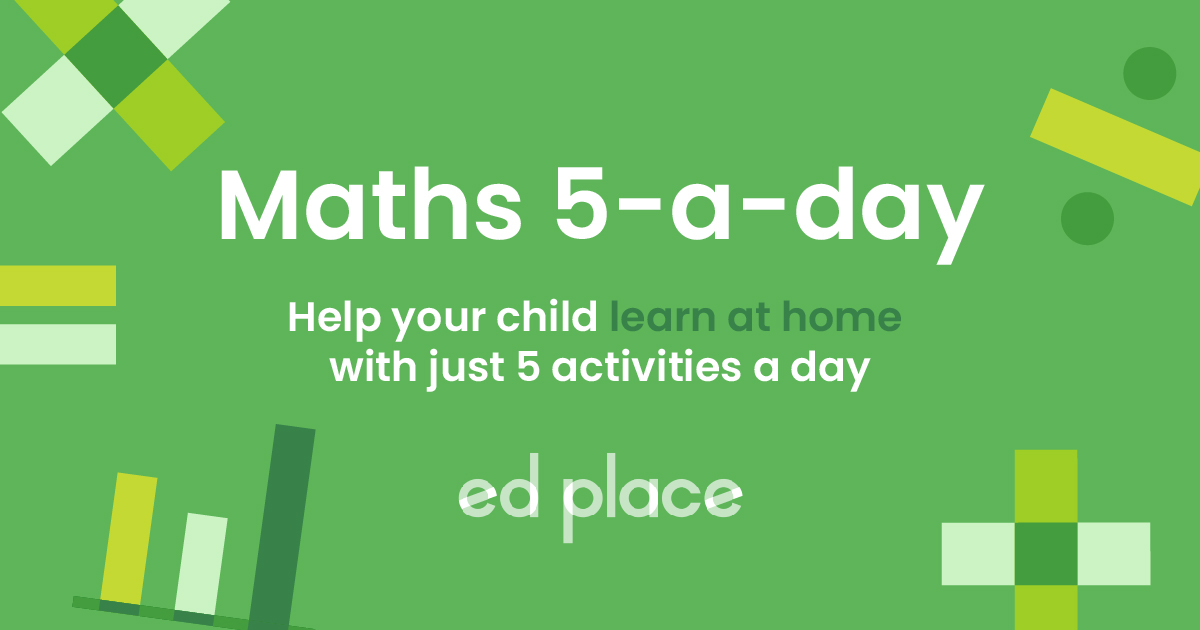# EdPlace's Year 2 Home Learning Maths Lesson: Subtraction

Looking for short lessons to keep your child engaged and learning? Our experienced team of teachers have created English, maths and science lessons for the home, so your child can learn no matter where they are.  And, as all activities are self-marked, you really can encourage your child to be an independent learner. Get them started on the lesson below and then jump into our teacher-created activities to practice what they've learnt. We've recommended five to ensure they feel secure in their knowledge - 5-a-day helps keeps the learning loss at bay (or so we think!).

Are they keen to start practising straight away? Head to the bottom of the page to find the activities.

Now...onto the lesson!

Key Stage 1 Statutory Requirements for Maths
Year 2 students should be able to add and subtract numbers using concrete objects, pictorial representations, and mentally, including a two-digit number and ones, a two-digit number and tens, two two-digit numbers.

# Wondering why your child's year 2 subtraction seems so complicated?

In schools today, children do not tend to be taught the ‘traditional’ addition and subtraction methods until year 3. Prior to this, there are many methods taught which mostly focus on understanding the foundations of subtraction. It is integral that your child understands the value of a number, which is why many methods for subtraction in year 2 are focused around place value.

At EdPlace, we are here to help you understand this method giving a step-by-step approach. The place value methods do vary slightly from school to school but the general understanding is the same. Every single lesson taught in school has an objective that each child should achieve. We are confident that by the end of reading this you and your child will:

1) Understand how to use place value drawing to complete subtraction questions

2) Apply this knowledge to answer an array of subtraction questions

3) Explain it back to you.

## Step 1 - Understand key terminology

Just like in school, it's important to go through the meaning of the vocabulary that will be used within the lesson so children understand what it all means!

Digits - a numeral from 0 - 9.

Place value - the value of each digit in a number. For example, 55 has 2 of the same digits but they each have a different value.

Tens - the digits that are worth ten, so in 53, the 5 is worth 5 tens, therefore, the value of this 5 is 50, not 5.

Ones - these used to be called units (and still are sometimes.) These are the digits that are worth one, so in 53, the 3 is worth 3 ones therefore the value of this 3 is 3.

Subtraction - to take away.

## Step 2 - Representing values in subtraction questions

We need to be able to represent the first number in the subtraction question in a place value chart before we tackle the question. Below is the number 35 drawn in a place value chart - they can be represented in slightly different ways so here are 3 examples of how they may be drawn.## Step 3 - Using place value charts to subtract

Now, we can use our number, represented in the place value chart. and look at the number we are subtracting or ‘taking away’.

Example

35 - 2 =

First, we look at our place value chart. We know that we need to take away 2 from 53, meaning that we just need to take away 2 ones on our place value chart. We can simply cross these out:We can now clearly see that the number left is 3 tens and 3 ones, or 33.

35 - 2 =  33

Now, we will look at taking away tens from a two-digit number:

47 - 20 = ?

For this question, we need to take away two tens. This would be drawn and represented as below:We now have 2 tens and 7 ones left which gives us the answer 27. Therefore, 47 – 20 = 27.

When we subtract a two-digit number from a two-digit number we do exactly the same as above but we take away both tens and ones from the number. When we cross the tens barrier it can get a bit more tricky. For example, if we want to calculate 53 - 8 we cannot just take away the ones here as there are only 3 ones and we need to take away 8. So instead, we take one of the tens and change them into ones. We take a ten away from the tens column by crossing it out and we put into the ones column as ten ones. There will now be 13 ones in the ones column (as there was already 3 there) and then, we can take away the 8 ones.So we now have 4 tens and 5 ones left. Therefore 53 – 8 = 45.

## Step 4 - Putting it into practise...

Now you could have a go at applying the above to answer these subtraction questions:

1) 27 - 5 =

2) 44 - 20 =

3) 49 - 16 =

4) 61 - 7 =

## Step 5 - Give it a go...

Now that you’ve covered this lesson together, why not put this to the test and assign your child the following subtraction activities in this order? All activities are created by teachers and automatically marked. Plus, with an EdPlace subscription, we can automatically progress your child at a level that's right for them. Sending you progress reports along the way so you can track and measure progress, together - brilliant!

1) 22

2) 24

3) 33

4) 54

Keep going! Looking for more activities, different subjects or year groups?

Click the button below to view the EdPlace English, maths, science and 11+ activity library